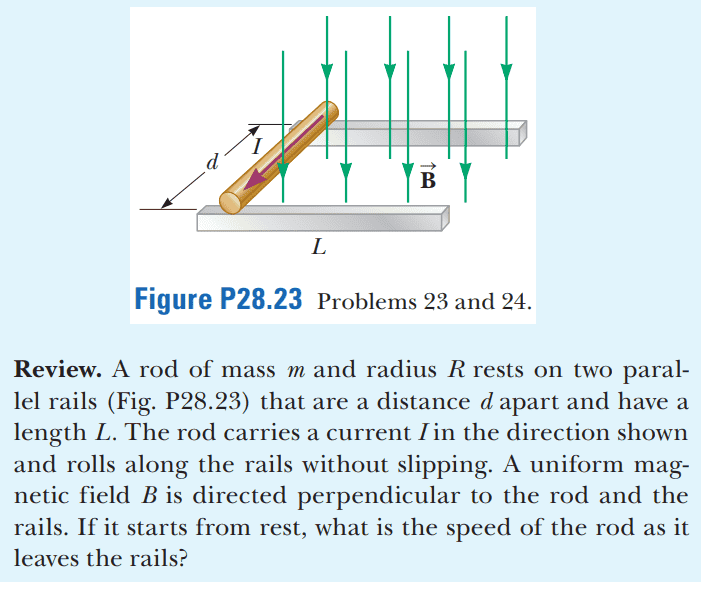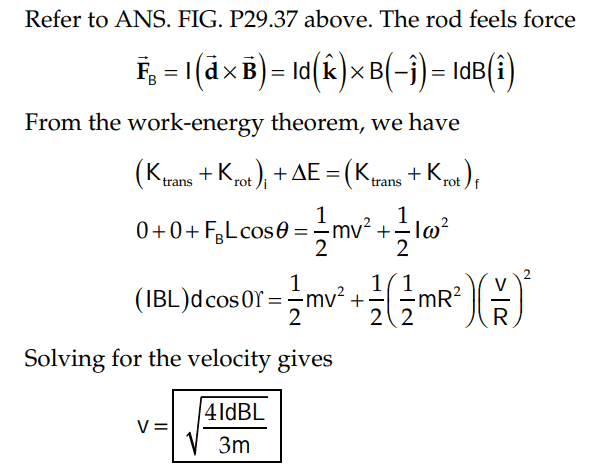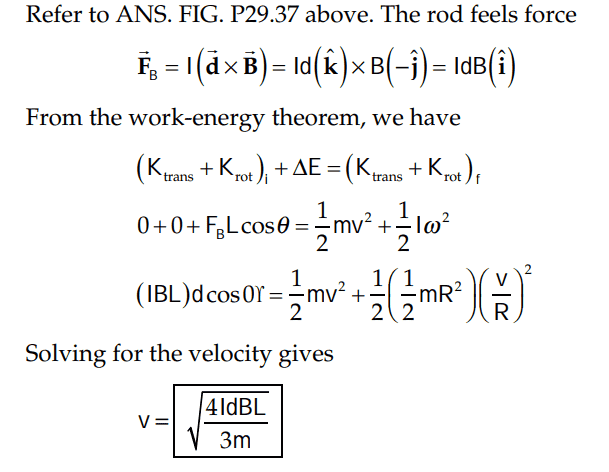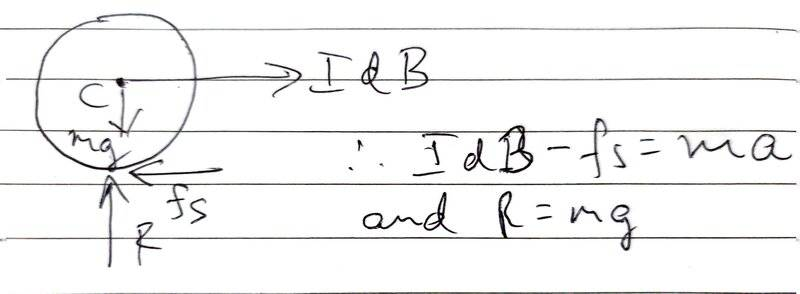# Why do we need conservation of energy for a rod accelerating due to magnetic force?

Callumnc1
Homework Statement:
Relevant Equations:
For this problem,Is the length vector into or out of the page and how do you tell?

EDIT: Why must we use conservation of energy for this problem? I tried solving it like this:
##IdB\sin90 = ma ##
##IdB = ma ##
##v_f = (2aL)^{1/2} ##
##v_f = (\frac {2dIBL} {m})^{1/2} ##

Which is incorrect according to solutions:Many thanks!

Last edited:

Homework Helper
Gold Member
2022 Award
Is the length vector into or out of the page and how do you tell?
Which length, L or d?
L is across, neither into the page nor out of it.
What formula do you plan to use?

•Callumnc1
Callumnc1
Which length, L or d?
L is across, neither into the page nor out of it.
What formula do you plan to use?
Thanks for your reply @haruspex ! I was refering to the length d. I plan to use the formula ## F_B = ILB\sin\theta ##

Thanks!

Callumnc1
Nevermind @haruspex , the solution uses the cross-product definition of magnetic forceI did't realise this was a conservation of energy problem. Why can't this be solved this way like I tried to do:

##IdB\sin90 = ma ##
##IdB = ma ##
##v_f = (2aL)^{1/2} ##
##v_f = (\frac {2dIBL} {m})^{1/2} ##

I guess it is because the force not only accelerates the center of mass but also rotates it (somehow?). I think they say assume rolling without slipping because otherwise not all of the work done by the magnetic force would go into kinetic energies of the rod.

Also how did they find ## I ## for the rod?

Last edited:
Homework Helper
Gold Member
2022 Award
because the force not only accelerates the center of mass but also rotates it
Yes.
rolling without slipping because otherwise not all of the work done by the magnetic force would go into kinetic energies of the rod
Or it could have been sliding without friction.
how did they find I for the rod?
I assume you mean the moment of inertia, not the current.
Same as for a disc. You can think of it as just a lot of discs stuck together.

•Callumnc1
Callumnc1
Yes.

Or it could have been sliding without friction.
Thanks for your reply @haruspex! How would it have ## K_{rot}## is it is sliding? It does not seem that their solution takes that into account, only rolling without slipping since they assume that the rod has and ##\omega##

I assume you mean the moment of inertia, not the current.
Same as for a disc. You can think of it as just a lot of discs stuck together.
Yes thanks you are correct. Thats a very insightful way to think about the moment of inertia of a rod!

Many thanks!

Homework Helper
Gold Member
2022 Award
How would it have ## K_{rot}## is it is sliding?
No, I meant that if it were sliding without friction instead of rolling then it would still be the case that all the work done goes into KE.

•Callumnc1
Callumnc1
No, I meant that if it were sliding without friction instead of rolling then it would still be the case that all the work done goes into KE.
Got it! So all the work goes into ##K_{trans} ## when sliding without friction. Thanks for your help!

vcsharp2003

For this problem,
View attachment 321150

Is the length vector into or out of the page and how do you tell?

EDIT: Why must we use conservation of energy for this problem? I tried solving it like this:
##IdB\sin90 = ma ##
##IdB = ma ##
##v_f = (2aL)^{1/2} ##
##v_f = (\frac {2dIBL} {m})^{1/2} ##

Which is incorrect according to solutions:
View attachment 321157

Many thanks!
In my view, the reason your initial attempt didn't give the correct answer is because you didn't consider the force of static friction which is a self-adjusting force and acts in rolling without slippage situations.The free body diagram of the rolling rod as it accelerates along the rails is as above; the equations of motion would then be different from the one you got in your attempted solution.

Note that rails cannot be smooth and frictionless as then the rod would not roll but slip on the rails.

The force shown as ##f_s## is the self-adjusting force of static friction and will always be such that ##f_s \le \mu_{s} \times R##. Also, when determining the magnetic force on the current carrying conductor, ##\vec {l}## direction must be taken in direction of conventional current ##I##.

If you knew the value as well as direction of ##f_s## then you could have used equation of motion to determine ##a## and then ##v_f## of the center of mass of the rolling rod, and there would be no need to use work-energy theorem/conservation of energy to solve this problem.

Work energy theorem states that ## W_{net} = \Delta {KE}## for any situation.

Last edited:
•Callumnc1
Callumnc1
In my view, the reason your initial attempt didn't give the correct answer is because you didn't consider the force of static friction which is a self-adjusting force and acts in rolling without slippage situations.

View attachment 321161

The free body diagram of the rolling rod as it accelerates along the rails is as above; the equations of motion would then be different from the one you got in your attempted solution.

Note that rails cannot be smooth and frictionless as then the rod would not roll but slip on the rails.

The force shown as ##f_s## is the self-adjusting force of static friction and will always be such that ##f_s \le \mu_{s} \times R##. Also, when determining the magnetic force on the current carrying conductor, ##\vec {l}## direction must be taken in direction of conventional current ##I##.

If you knew the value as well as direction of ##f_s## then you could have used equation of motion to determine ##a## and then ##v_f## of the center of mass of the rolling rod, and there would be no need to use work-energy theorem/conservation of energy to solve this problem.

Work energy theorem states that ## W_{net} = \Delta {KE}## for any situation.
Thank very much for those insights! That is very interesting!

•vcsharp2003
vcsharp2003
Thank very much for those insights! That is very interesting!
If you wanted to solve this problem without using conservation of energy then you could do so by writing the correct equation of motion and also writing the torque equation for the rolling object about an axis through object's center of mass or object's point of contact with the rails.

The torque equation is ##\tau = I \times \alpha## and the equation of motion is ##F= m \times a##. ##I## i.e. moment of inertia of rolling object , must be taken about the same axis as the torque ##\tau##.

(##\alpha## is angular acceleration of the rolling object and is related to linear acceleration ##a## according to the equation ##a= \alpha \times r##)

•Callumnc1
Callumnc1
If you wanted to solve this problem without using conservation of energy then you could do so by writing the correct equation of motion and also writing the torque equation for the rolling object about an axis through object's center of mass or object's point of contact with the rails.

The torque equation is ##\tau = I \times \alpha## and the equation of motion is ##F= m \times a##. ##I## i.e. moment of inertia of rolling object , must be taken about the same axis as the torque ##\tau##.

(##\alpha## is angular acceleration of the rolling object and is related to linear acceleration ##a## according to the equation ##a= \alpha \times r##)
Thanks for pointing that out @vcsharp2003 ! I should try that sometime!

Homework Helper
Gold Member
2022 Award
It does not seem that their solution takes that into account, only rolling without slipping since they assume that the rod has and
The problem text says "rolls without slipping" so why solve the problem in a completely different situation?

•Callumnc1
Homework Helper
Gold Member
2022 Award
Thank you for your reply @malawi_glenn ! Yeah true, my curiosity sometimes extends beyond the problem :)
•